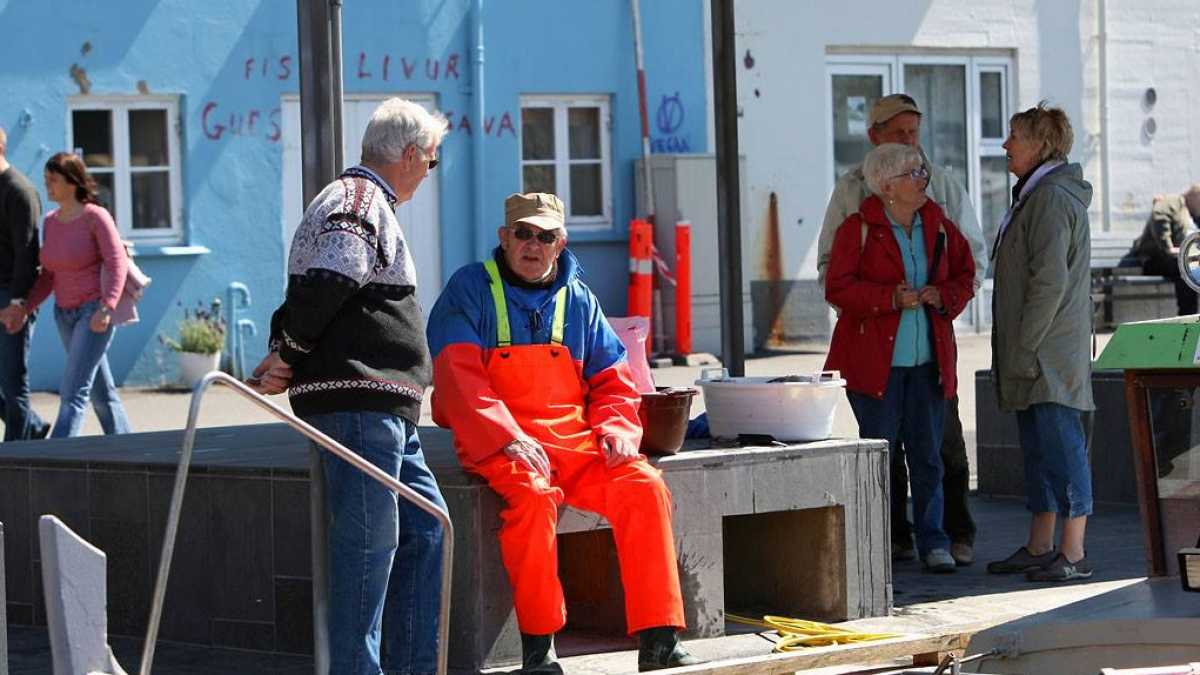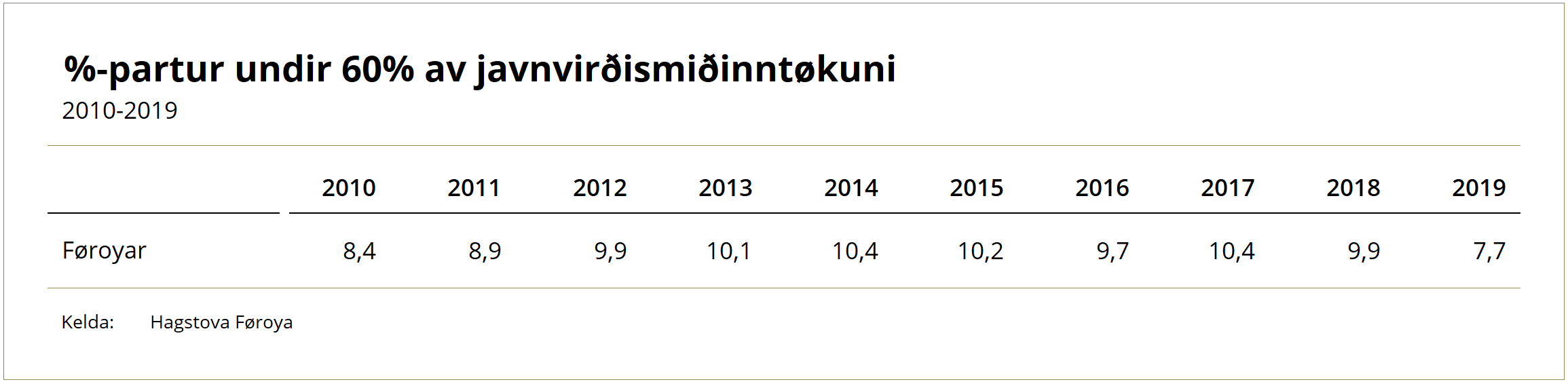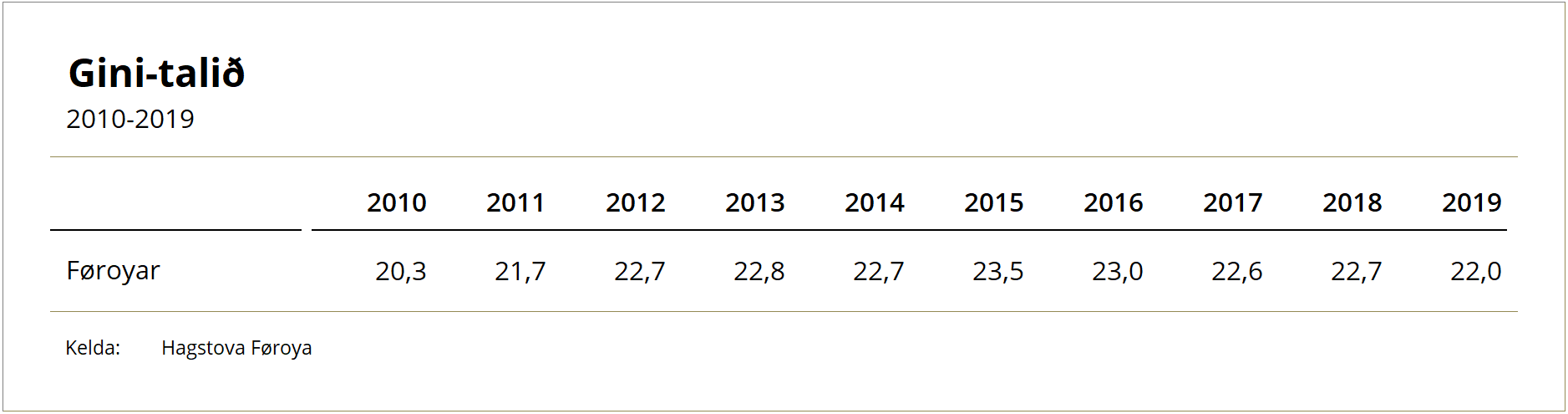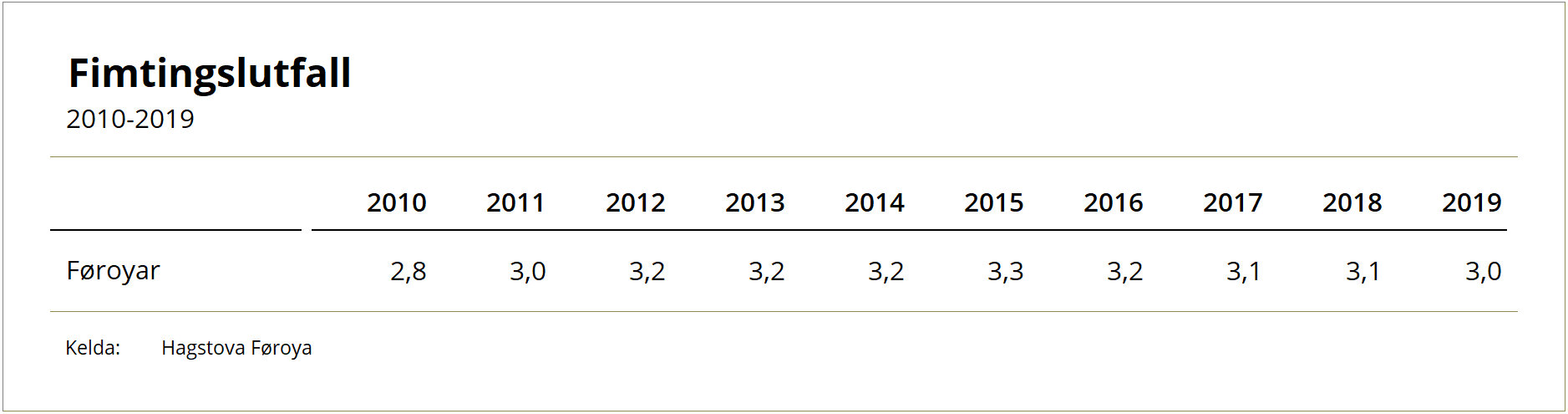# 12. Nov 2021 A more equal income distribution and fewer people at risk of poverty in 2019

The latest income figures reveal a relatively big drop in the number of people at risk of poverty in 2019 compared to previous years. The income distribution in the Faroe Islands has also become more equal.Income statistics have been updated in the statbank up to and including 2019.

The statistics outline the spending potential of Faroese households and individuals, including information on the number of people below a certain income mark and the income distribution across the population. There also tables showing the number of people in each income bracket

## Relatively fewer people at risk of poverty

The at-risk-of-poverty rate is an international measurement which counts the share of the population with the lowest relative spending potential. These statistics are based on the equivalent income, also known as ‘equivalised disposable income’, which is an equivalised household income figure adjusted for different household compositions. Read more about equivalent incom.

The at-risk-of-poverty rate indicates the proportion of people with an equivalent income below 60% of the national median equivalent income.

##The table above reveals a 2.2% drop from 2018 to 2019. This is predominantly due to the 67+ age group, many of whom (at times up to almost 30%) have been below the at-risk-of-poverty threshold, moving beyond this threshold in 2019. It should be noted that a large proportion of this age group has been close to the threshold since these figures were first compiled. About 9.4% fewer 67+ citizens were below the threshold in 2019 compared to 2018. The statistics also give a general indication that larger households have surpassed the threshold, while the figure for 67+ citizens living alone remains relatively unchanged, with more than half of this demographic living below the at-risk-of-poverty threshold.

The table above shows the total number of people below the at-risk-of-poverty threshold across the Faroe Islands. However, the figures and trends vary across the different demographics. More detailed figures are available in the statbank.

## Gini coefficient and income quintile ratio: a more equal income distribution

The Gini coefficient and the income quintile ratio (also known as the ‘S80/S20 ratio’) are two of the metrics used for describing a nation’s income distribution.

The Gini coefficient is used as a measure of income inequality. If all citizens in a country have the same income, the country’s income Gini coefficient would be 0. If one household earns all of the country’s income, the income Gini coefficient would be 100. In 2019, the Gini coefficient was 22.0, compared to 22.7 in 2018.The income quintile ratio (80/20) divides the population into five equally large groups (quintiles) and compares the total income of the 20% of the population with the highest income (top quintile) to that of the 20% with the lowest income (bottom quintile). In 2019, the top quintile had an income three times higher than the bottom quintile. In 2018, this ratio was 3.1.The tables above show the Gini coefficient and the income quintile ratio for the Faroe Islands.

More detailed figures are available in the statbank.